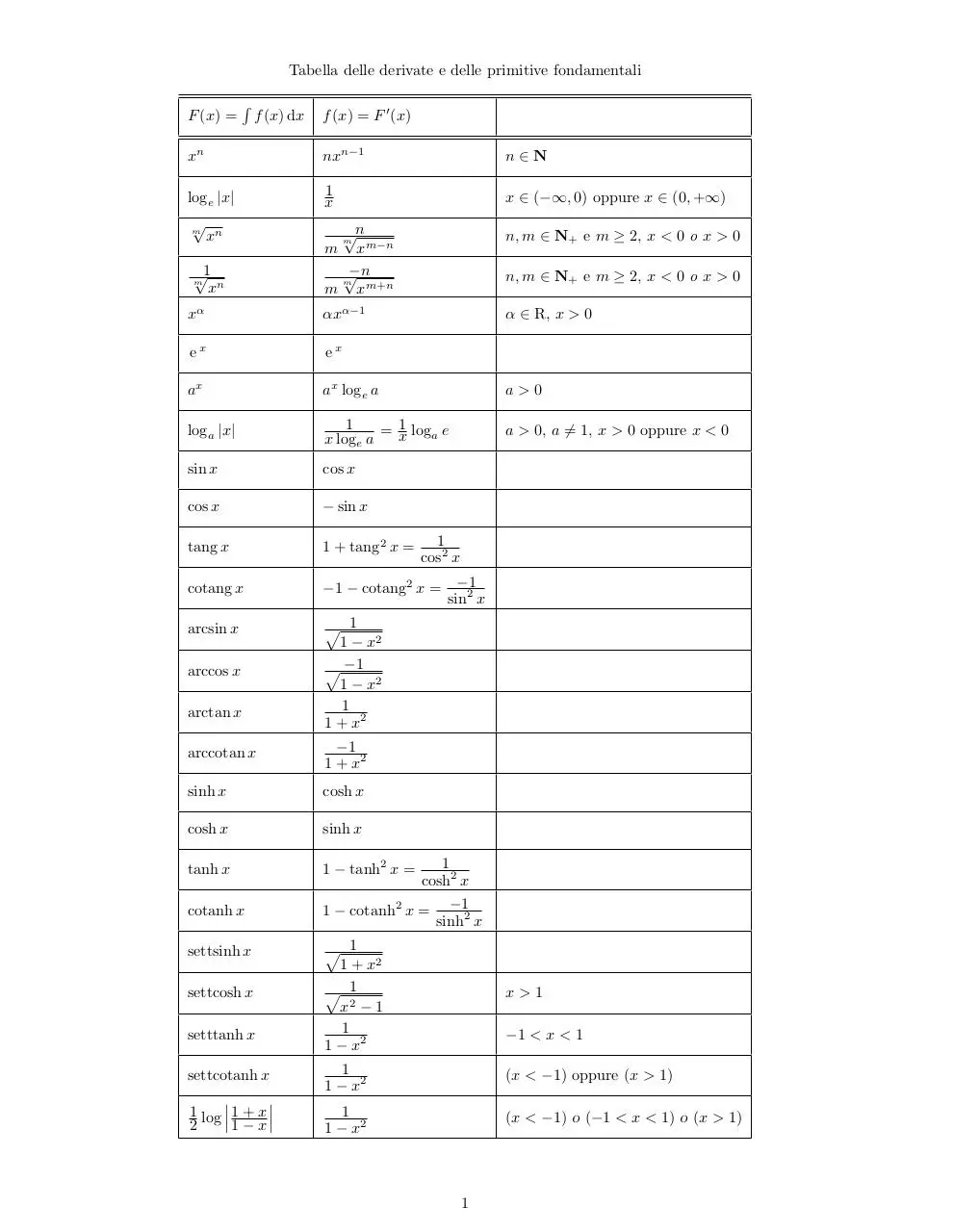# derivate fondamentali .pdf

### File information

This PDF 1.2 document has been generated by TeX output 2003.09.25:1444 / dvipdfm 0.13.2c, Copyright Â© 1998, by Mark A. Wicks, and has been sent on pdf-archive.com on 29/11/2014 at 16:42, from IP address 151.29.x.x. The current document download page has been viewed 16112 times.
File size: 52.83 KB (1 page).
Privacy: public file

### Document preview

Tabella delle derivate e delle primitive fondamentali
F (x) =

R

f (x) dx

f (x) = F 0 (x)

xn

nxn−1

n∈N

loge |x|

1
x

x ∈ (−∞, 0) oppure x ∈ (0, +∞)

√n
xm−n
√−n
m
m xm+n

n, m ∈ N+ e m ≥ 2, x &lt; 0 o x &gt; 0

αxα−1

α ∈ R, x &gt; 0

ex

ex

ax

ax loge a

a&gt;0

loga |x|

1
1 log e
=x
a
x loge a

a &gt; 0, a 6= 1, x &gt; 0 oppure x &lt; 0

sin x

cos x

cos x

− sin x

tang x

1 + tang2 x =

cotang x

−1 − cotang2 x = −1
sin2 x

arcsin x

p 1
1 − x2
p −1
1 − x2
1
1 + x2

m

xn

√1
xn

m

arccos x
arctan x

m

m

n, m ∈ N+ e m ≥ 2, x &lt; 0 o x &gt; 0

1
cos2 x

arccotan x

−1
1 + x2

sinh x

cosh x

cosh x

sinh x

tanh x

1 − tanh2 x =

cotanh x

1 − cotanh2 x =

settsinh x

p 1
1 + x2
p 1
x2 − 1
1
1 − x2

x&gt;1

1
1 − x2

(x &lt; −1) oppure (x &gt; 1)

1
1 − x2

(x &lt; −1) o (−1 &lt; x &lt; 1) o (x &gt; 1)

settcosh x
setttanh x
settcotanh x
¯
¯
1 log ¯¯ 1 + x ¯¯
2
1−x

1
cosh2 x
−1
sinh2 x

−1 &lt; x &lt; 1

1derivate fondamentali.pdf (PDF, 52.83 KB)

### Share on social networks

#### HTML Code

Copy the following HTML code to share your document on a Website or Blog